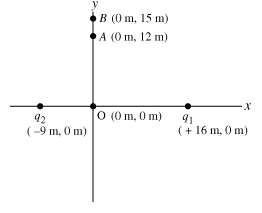# Problem: Two point charges are placed on the x axis. The first charge, q1 = 8.00 nC, is placed a distance 16.0 m from the origin along the positive x-axis; the second charge, q2 = 6.00 nC, is placed a distance 9.00 m from the origin along the negative x-axis.Calculate the electric field at point A, located at coordinates (0 m, 12.0 m).Give the x and y components of the electric field as an ordered pair.Express your answer in newtons per coulomb to three significant figures.EAx, EAy =

###### FREE Expert Solution

Electric field:

$\overline{){\mathbf{E}}{\mathbf{=}}\frac{\mathbf{k}\mathbf{q}}{{\mathbf{r}}^{\mathbf{2}}}}$

The electric field at point A is given by:

EA = Eq1 + Eq2

The electric field generated by q1:

r1 = sqrt(x2 + y2)

r1 = sqrt(162 + 122) = 20 m

85% (177 ratings)###### Problem Details

Two point charges are placed on the x axis. The first charge, q1 = 8.00 nC, is placed a distance 16.0 m from the origin along the positive x-axis; the second charge, q2 = 6.00 nC, is placed a distance 9.00 m from the origin along the negative x-axis.

Calculate the electric field at point A, located at coordinates (0 m, 12.0 m).

Give the x and y components of the electric field as an ordered pair.

EAx, EAy =